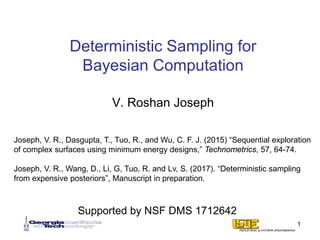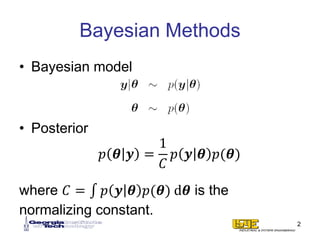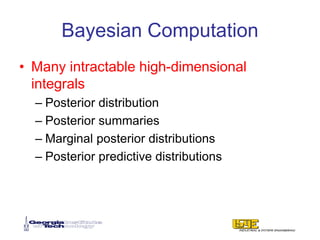Diese Präsentation wurde erfolgreich gemeldet.

# Program on Quasi-Monte Carlo and High-Dimensional Sampling Methods for Applied Mathematics Opening Workshop, Deterministic Sampling for Bayesian Computation - Roshan Vengazhiyil, Aug 31, 2017

Anzeige
Anzeige
Anzeige
Anzeige
Anzeige
Anzeige
Anzeige
Anzeige
Anzeige
Anzeige
Anzeige×

1 von 47 Anzeige

# Program on Quasi-Monte Carlo and High-Dimensional Sampling Methods for Applied Mathematics Opening Workshop, Deterministic Sampling for Bayesian Computation - Roshan Vengazhiyil, Aug 31, 2017

Markov chain Monte Carlo (MCMC) methods are popularly used in Bayesian computation. However, they need large number of samples for convergence which can become costly when the posterior distribution is expensive to evaluate. Deterministic sampling techniques such as Quasi-Monte Carlo (QMC) can be a useful alternative to MCMC, but the existing QMC methods are mainly developed only for sampling from unit hypercubes. Unfortunately, the posterior distributions can be highly correlated and nonlinear making them occupy very little space inside a hypercube. Thus, most of the samples from QMC can get wasted. The QMC samples can be saved if they can be pulled towards the high probability regions of the posterior distribution using inverse probability transforms. But this can be done only when the distribution function is known, which is rarely the case in Bayesian problems. In this talk, I will discuss a deterministic sampling technique, known as minimum energy designs, which can directly sample from the posterior distributions.

Markov chain Monte Carlo (MCMC) methods are popularly used in Bayesian computation. However, they need large number of samples for convergence which can become costly when the posterior distribution is expensive to evaluate. Deterministic sampling techniques such as Quasi-Monte Carlo (QMC) can be a useful alternative to MCMC, but the existing QMC methods are mainly developed only for sampling from unit hypercubes. Unfortunately, the posterior distributions can be highly correlated and nonlinear making them occupy very little space inside a hypercube. Thus, most of the samples from QMC can get wasted. The QMC samples can be saved if they can be pulled towards the high probability regions of the posterior distribution using inverse probability transforms. But this can be done only when the distribution function is known, which is rarely the case in Bayesian problems. In this talk, I will discuss a deterministic sampling technique, known as minimum energy designs, which can directly sample from the posterior distributions.

Anzeige
Anzeige

### Program on Quasi-Monte Carlo and High-Dimensional Sampling Methods for Applied Mathematics Opening Workshop, Deterministic Sampling for Bayesian Computation - Roshan Vengazhiyil, Aug 31, 2017

1. 1. Deterministic Sampling for Bayesian Computation V. Roshan Joseph 1 Joseph, V. R., Dasgupta, T., Tuo, R., and Wu, C. F. J. (2015) “Sequential exploration of complex surfaces using minimum energy designs,” Technometrics, 57, 64-74. Joseph, V. R., Wang, D., Li, G, Tuo, R. and Lv, S. (2017). “Deterministic sampling from expensive posteriors”, Manuscript in preparation. Supported by NSF DMS 1712642
2. 2. Bayesian Methods • Bayesian model • Posterior where 𝐶𝐶 = ∫ 𝑝𝑝 𝒚𝒚 𝜽𝜽 𝑝𝑝(𝜽𝜽) d𝜽𝜽 is the normalizing constant. 2 𝑝𝑝 𝜽𝜽 𝒚𝒚 = 1 𝐶𝐶 𝑝𝑝 𝒚𝒚 𝜽𝜽 𝑝𝑝(𝜽𝜽)
3. 3. Bayesian Computation • Many intractable high-dimensional integrals – Posterior distribution – Posterior summaries – Marginal posterior distributions – Posterior predictive distributions
4. 4. Markov Chain Monte Carlo Methods • Metropolis et al. 1953, Hastings 1970, Geman and Geman 1984, Gelfand and Smith 1990, …
5. 5. An Example 5
6. 6. MCMC • Metropolis Algorithm: 6
7. 7. Metropolis Algorithm 7
8. 8. Random Sample 8
9. 9. Disadvantages of MCMC • 𝑓𝑓(𝒙𝒙) may be expensive and time consuming to evaluate. • 𝑔𝑔(𝒙𝒙) may be expensive and time consuming to evaluate. 9 Simulation: Integration: 𝒙𝒙𝑖𝑖~𝑓𝑓 𝒙𝒙 , 𝑖𝑖 = 1, … , 𝑛𝑛
10. 10. Simulation problem Hung, Joseph, Melkote (2009) Expensive
11. 11. Integration problem Uncertainty sources Input Output Propagation of uncertainty 4 11 • Support points: Simon Mak’s talk on Tuesday
12. 12. Two Possible Solutions 1. Approximate 𝑓𝑓(𝑥𝑥) using an easy-to- evaluate surrogate model ̂𝑓𝑓(𝑥𝑥) and generate MCMC sample using ̂𝑓𝑓(𝑥𝑥). – High dimensional function approximation is hard! 2. Use a deterministic sample that is well- spaced instead of a random sample. – QMC 12
13. 13. Deterministic Sample • Quasi-Monte Carlo (QMC): 50-point Sobol sequence 13
14. 14. Deterministic Sample • Quasi-Monte Carlo (QMC): 50-point Sobol sequence 14
15. 15. Transformation to the Unit Hypercube • “We only need to consider point sets in [0,1]𝑝𝑝 , otherwise transform using inverse distribution function”. • If 𝑥𝑥1, … , 𝑥𝑥𝑝𝑝are independent with distribution functions 𝐹𝐹1, … , 𝐹𝐹𝑝𝑝, then transform a uniform sample 𝑢𝑢1, … , 𝑢𝑢𝑝𝑝 using 𝐹𝐹1 −1 𝑢𝑢1 , … , 𝐹𝐹𝑝𝑝 −1 𝑢𝑢𝑝𝑝 . 15
16. 16. Limitations in Bayesian problems • 𝑥𝑥1, … , 𝑥𝑥𝑝𝑝 are rarely independent. • Joint density is known only up to a proportionality constant: – Distribution function is unknown. – Inverse distribution function is unknown. – So this rarely works! 16
17. 17. Another recommended strategy • Use an importance sampling density whose inverse distribution function can be easily obtained. • However, finding an importance sampling density in a Bayesian problem is very hard. – So this rarely works! 17
18. 18. Research Problem • How to generate a deterministic sample directly from a probability density that is known only up to a proportionality constant? 18
19. 19. Minimum Energy Designs • Experimental region: • Experimental design: – View the n points as charged particles inside a box. – They will occupy positions that will minimize the total potential energy. 19
20. 20. MED-continued • Let q(xi) be the charge at xi. • Then, minimize 20
21. 21. Charge function-Intuition • Charge should be inversely proportional to density value. 21
22. 22. Generalized MED • As 22
23. 23. Limiting distribution 23 Theorem: There exists a probability measure 𝑃𝑃 such that 𝑃𝑃𝑛𝑛 converges to 𝑃𝑃. Moreover, 𝑃𝑃 has a density 𝑓𝑓 over 𝑋𝑋 with 𝑓𝑓 𝒙𝒙 ∝ 1 𝑞𝑞2𝑝𝑝 𝒙𝒙 .
24. 24. Charge Function • So if we choose the charge function to be then we can obtain the target distribution. 24
25. 25. Interpretation 25
26. 26. Probability Balancing 26
27. 27. Sphere Packing Problems • Minimum Riesz energy points – Borodachov, Hardin, Saff (2008a,b) 27
28. 28. Uniform Distribution • MED for 𝑛𝑛 = 25, 𝑝𝑝 = 2 • The effective sample size for each dimension is only 𝑛𝑛1/𝑝𝑝 . 28
29. 29. Generalized Distance 29
30. 30. Choice of s 30 MaxPro Low discrepancy and good space-filling!
31. 31. MaxPro Design 31 Joseph, V. R., Gul, E., and Ba, S. (2015). “Maximum Projection Designs for Computer Experiments,” Biometrika, 102, 371-380.
32. 32. Probability Balancing 32
33. 33. A Greedy Algorithm • It can get stuck in a local optimum, but good designs are produced with a good starting point: • Requires a global optimization at each step-> Computationally very expensive! 33
34. 34. Complex probability distributions where C is the (unknown ) normalizing constant. C is not needed! 𝑓𝑓 𝑥𝑥 = 1 𝐶𝐶 ℎ(𝑥𝑥) 34
35. 35. Bayesian Computation 35
36. 36. 36
37. 37. 37
38. 38. Computational time & Number of evaluations • Global optimization using Generalized simulated annealing (GSA) 38
39. 39. A New Algorithm 39 𝑓𝑓(𝑥𝑥)𝛾𝛾 Tempering: 𝛾𝛾 = 0 𝑛𝑛 QMC points in [0,1]𝑝𝑝 𝛾𝛾 = 1/(𝐾𝐾 − 1) 𝑛𝑛 MED points out of 2𝑛𝑛 points … 𝛾𝛾 = 1 𝑛𝑛 MED points out of K𝑛𝑛 points
40. 40. 40
41. 41. 41
42. 42. 42
43. 43. 43
44. 44. 44
45. 45. 45 #evaluations=763
46. 46. Computational time & Number of evaluations 46 21 hours 0.5 hours
47. 47. Conclusions 47 0 Cost of evaluations MCMC QMC+ MCMC QMC+ Function approximation +MCMC